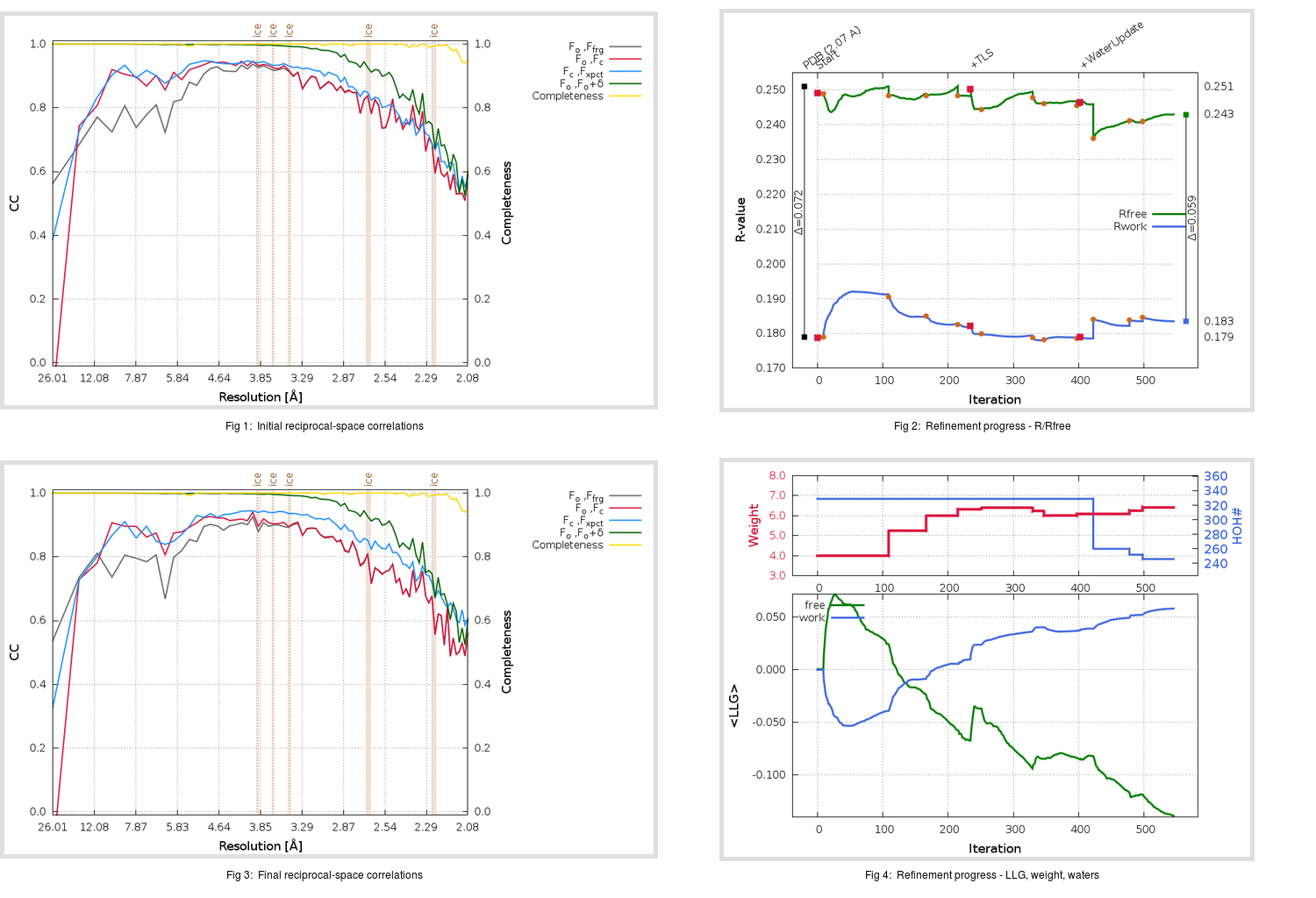Content:

```    Diffraction limits & principal axes of ellipsoid fitted to diffraction cut-off surface:
1.992         0.9964   0.0000  -0.0853       0.993 a* - 0.120 c*
1.946         0.0000   1.0000   0.0000       b*
2.272         0.0853   0.0000   0.9964       0.219 a* + 0.976 c*
```

## Deposited

` `
 Date deposited Date data collection Resolution R, Rfree 20200315 20200304 2.07 0.1750 0.2510

Molprobity (CCP4 7.0 version) summary:

```Ramachandran outliers =   0.66 %
favored =  96.36 %
Rotamer outliers      =   0.76 %
C-beta deviations     =     0
Clashscore            =   5.27
RMS(bonds)            =   0.0131
RMS(angles)           =   1.80
MolProbity score      =   1.52
Resolution            =   2.07
R-work                =   0.1750
R-free                =   0.2510
```

```Number of waters      =   329

<B> (all atoms) =   45.88 ( sd =   12.45 ) for       2735 non-hydrogen atoms
<B>   (protein) =   44.86 ( sd =   12.21 ) for       2370 non-hydrogen atoms
<B>     (water) =   52.80 ( sd =   11.21 ) for        329 non-hydrogen atoms
<B>    (others) =   49.66 ( sd =   20.51 ) for         36 non-hydrogen atoms

B min/max       (all non-hydrogen atoms) =   26.66 /  105.15
B min/max   (protein non-hydrogen atoms) =   26.66 /  103.42
B min/max     (water non-hydrogen atoms) =   26.90 /  105.15
B min/max     (other non-hydrogen atoms) =   37.65 /   72.68
```

## BUSTER (re-)refinement

` `

Molprobity (CCP4 7.0 version) summary:

```Ramachandran outliers =   0.00 %
favored =  97.02 %
Rotamer outliers      =   1.89 %
C-beta deviations     =     0
Clashscore            =   3.37
RMS(bonds)            =   0.0120
RMS(angles)           =   1.56
MolProbity score      =   1.51
Resolution            =   2.07
R-work                =   0.1835
R-free                =   0.2430
```

```Number of waters      =   246

<B> (all atoms) =   42.88 ( sd =    9.50 ) for       2652 non-hydrogen atoms
<B>   (protein) =   42.04 ( sd =    8.93 ) for       2370 non-hydrogen atoms
<B>     (water) =   50.57 ( sd =    9.45 ) for        246 non-hydrogen atoms
<B>    (others) =   45.34 ( sd =   20.91 ) for         36 non-hydrogen atoms

B min/max       (all non-hydrogen atoms) =   24.36 /   80.11
B min/max   (protein non-hydrogen atoms) =   27.22 /   80.11
B min/max     (water non-hydrogen atoms) =   24.36 /   78.66
B min/max     (other non-hydrogen atoms) =   31.38 /   66.56
```

Refinement progression:Results:

` `
 File Remark 5REX_aB_refine.01_03_refine.pdb.gz exact refinement commands are in header 5REX_aB_refine.01_03_refine.mtz.gz including original deposited data and several re-refinement map coefficients 5REX_aB_refine.01_03_BUSTER_model.cif.gz including any non-standard compound restraints 5REX_aB_refine.01_03_BUSTER_refln.cif.gz